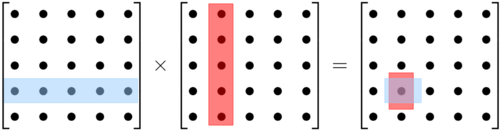## How to multiply matrices

This is for my homies in math class.

Mathematical matrices are blocks of numbers, arrayed in 2-D. (Higher-dimensional arrays are called tensors.)

1.Left “times” right equals target. Each entry in the target is the result of a series of +’s and ×’s along the red and blue. A long sum of pairwise products.
2. Your left hand goes across and your right hand goes up/down.where.
3. There need to be as many abcdefg’s as there are 1234567’s or else the operation can’t be done.
4. Also you can tell how big the output matrix will be. There can be three blue rows so the output has three rows. There can be four red columns so the output has four columns.
5. This is the “inner product” because multiplying vector-shaped blocks (tall blocks) like Aᵀ•B results in an equal or smaller sized output.

(There is also an “outer product” which is a different way of combining the info from the two matrices. That gives you an equal or larger shaped result when you multiply vector/list-shaped tall blocks A∧B.)

6. Try playing around with this one or that one.

Matrix multiplication is the simplest example of a linear operator, the broad class of which explains quantum mechanics and ODE’s. You can also apply different matrices at different points as in a vector field — on a flat surface or a curvy, holey surface.